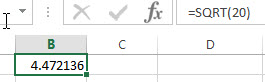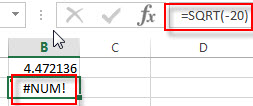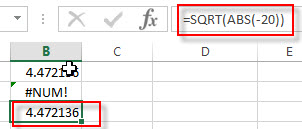## Excel SQRT Function

This post will guide you how to use Excel SQRT function with syntax and examples in Microsoft excel.

### Description

The Excel SQRT function returns the square root of a positive number. And if you provide a negative number, the function will return #NUM! Error.

The SQRT function is a build-in function in Microsoft Excel and it is categorized as a Math and Trigonometry Function.

The SQRT function is available in Excel 2016, Excel 2013, Excel 2010, Excel 2007, Excel 2011 for Mac.

### Syntax

The syntax of the SQRT function is as below:

`=SQRT (number)`

Where the SQRT function argument is:

• Number -This is a required argument. The number for which you want to calculate the square root.

Note:

• If the supplied number is negative, the SQRT function will return #NUM! Error.
• If the supplied number is non-numeric, The SQRT function will return #VALUE! Error.

### Excel SQRT Function Examples

The below examples will show you how to use Excel SQRT Function to calculate the square root for a positive number.

1# to calculate the square root of number 20, enter the following formula in Cell B1.

`=SQRT(20)`2# to calculate the square root of a negative number -20, enter the following formula in Cell B2.

`=SQRT(-20)`You will see that the #NUM! Error message is returned.

3# using the ABS function to get the absolute value of a negative number and then combine with the SQRT function to calculate the square root to avoid the #NUM! Error message. Enter the following formula in Cell B3.

`=SQRT(ABS(-20))`### Related Functions

• Excel ABS Function
The Excel ABS function returns the absolute value of a number.The ABS function is a build-in function in Microsoft Excel and it is categorized as a Math and Trigonometry Function.The syntax of the ABS function is as below:=ABS (number)…